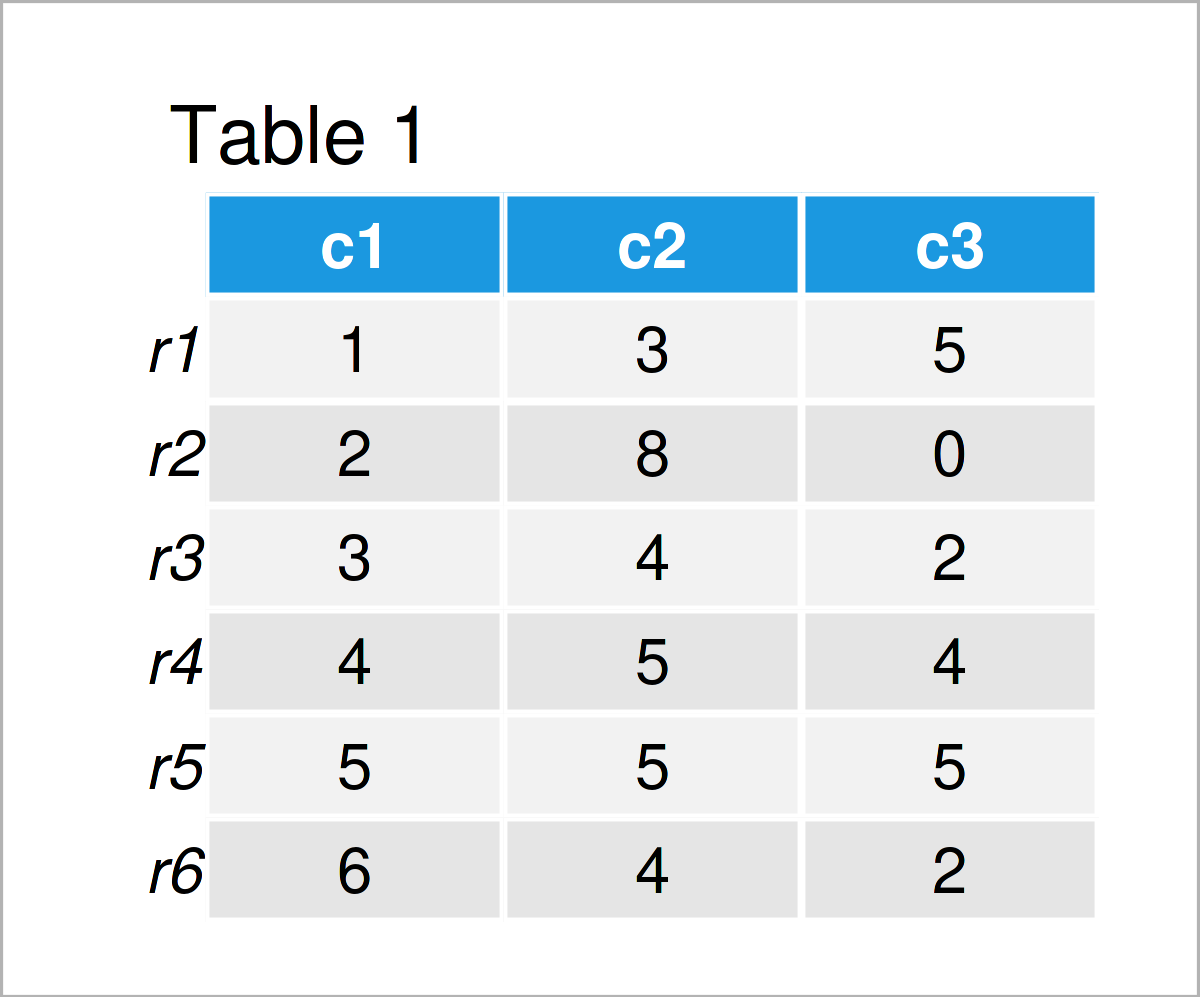# Get Row & Column Name of Min & Max in R (2 Examples)

In this R tutorial you’ll learn how to get row and column names of the min or max values.

The article is structured as follows:

Let’s dive right into the examples!

## Example Data

We’ll use the following data frame as a basement for this R programming language tutorial:

```data <- data.frame(c1 = 1:6,                            # Create example data
c2 = c(3, 8, 4, 5, 5, 4),
c3 = c(5, 0, 2, 4, 5, 2))
rownames(data) <- paste0("r", 1:nrow(data))
data                                                    # Print example data```Table 1 visualizes the RStudio console output and shows that the example data has six rows and three columns. The variable c1 has the integer class and the variables c2 and c3 have the numeric class.

## Example 1: Print Row & Column Names of Minimum Value in Data Frame

Example 1 demonstrates how to extract the row and column names of the minimum value in the example dataset.

For this task, the rownames(), which(), and min() functions are employed as demonstrated below.

First, let’s extract the row name.

```rowname_min <- rownames(data)[which(data == min(data),  # Extract row name of min
arr.ind = TRUE)[ , 1]]
rowname_min                                             # Print row name of min
#  "r2"```

Now let’s extract the column name.

```colname_min <- colnames(data)[which(data == min(data),  # Extract column name of min
arr.ind = TRUE)[ , 2]]
colname_min                                             # Print column name of min
#  "c3"```

Great we managed to return the row and column names of the minimum value. Now let’s move on to the maximum.

## Example 2: Print Row & Column Names of Maximum Value in Data Frame

The following R programming syntax explains how to find the row and column names of the maximum value in the sample dataset in R.

First, let’s get the row name.

```rowname_max <- rownames(data)[which(data == max(data),  # Extract row name of max
arr.ind = TRUE)[ , 1]]
rowname_max                                             # Print row name of max
#  "r2"```

Now let’s extract the name of the column as well.

```colname_max <- colnames(data)[which(data == max(data),  # Extract column name of max
arr.ind = TRUE)[ , 2]]
colname_max                                             # Print column name of max
#  "c2"```

Looks good!

Note: In the previous examples, we have applied our R syntax to a data frame. However, please note that we could apply the same code to a matrix object as well.

## Video, Further Resources & Summary

Do you need further info on the content of this tutorial? Then I recommend watching the following video on my YouTube channel. I’m explaining the content of this tutorial in the video:

Besides the video, you might want to have a look at the related tutorials on this website:

Summary: This post has demonstrated how to retrieve the row and column names of the min and max input in the dataset in R programming. Tell me about it in the comments section below, in case you have additional comments or questions.

This page was created in collaboration with Cansu Kebabci. Have a look at Cansu’s author page to get more information about her professional background, a list of all his tutorials, as well as an overview on her other tasks on Statistics Globe.

Subscribe to the Statistics Globe Newsletter